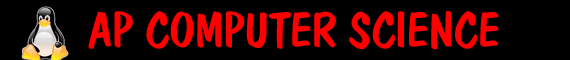Test#1 Review   Chapters Chpt 1,2,3 excluding section we did not cover (ie 2.10, 3.6)   Topics Chpt1: binary ideas of java comments reserved words types of errors Chpt2: objects and classes primitive data types Strings length() equals compareTo substring (int begin) substring(int begin, int end) ++, +=, ... Math class random pow abs sqrt import statement and packages Chpt 3: if else statements &&, || and ! comparing Strings (compareTo) comparing doubles and ints for, while loops The test will be both short answer: ``` public class Questions1_4 { public Questions1_4 { System.out.print("Here"); System.out.println("There " + "Everywhere"); System.out.println("But not" + "in Texas"); } } 1) The program will print the word "Here" and then print a) "There Everywhere" on the line after "Here" b) "There" on the line after "Here" and "Everywhere" on the line after "There" c) "There Everywhere" on the same line as "Here" d) "ThereEverywhere" on the same line as "Here" "ThereEverywhere" on the line after "Here" ``` Long answer (generally write psuedocode). In psuedocode- 2. Write a method called double findPosAvg(int x, int y, int z) that will find the average of the absolute values of x, y, and z and return that value. 3. Write a method called public double convertToFahr (int celsius) that will convert the temperature in celsisu to fahr. If it is below 32 it will prints "its cold' if its above 32 but below 60 it will says "thats mild" if its above 60 but below 85 it will print "thats nice" if its higher than 85 it will print "thats too hot". It will then return the converted temperature (the formula for conversion is fahr = 9/5celsius + 32 4. Write a method boolean isPrime(int x) that will return true if the number is prime, false otherwise. [this might be extra credit as requires for loops] 5. Write a method void cheer (string word) that will take a word (say apple) and print "Give me an a! Give me p! Give me p! Give me l! Give me e!